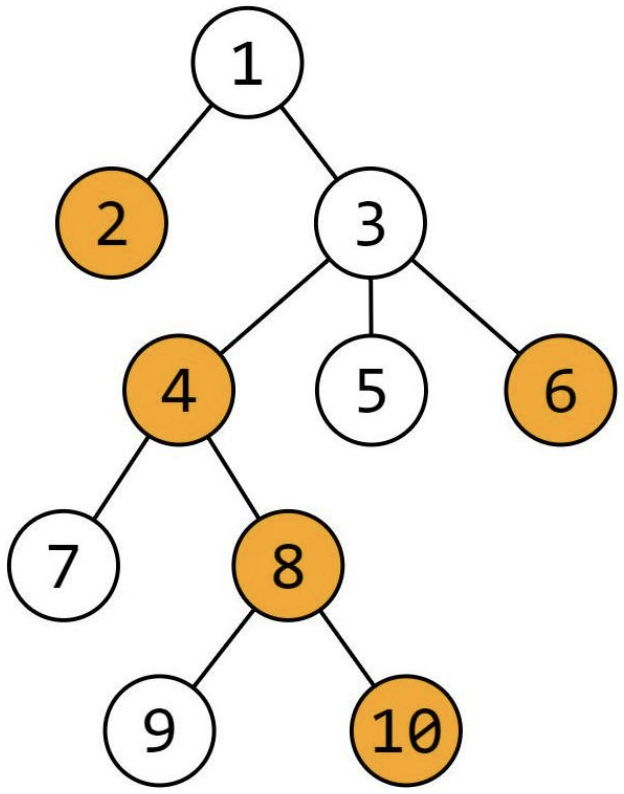시간 제한메모리 제한제출정답맞힌 사람정답 비율
2 초 1024 MB11000.000%

## 문제

Deni is the boss of a company with $N$ workers, numbered from $1$ to $N$. The company structure is strictly hierarchical – every worker (except number $1$) has exactly one direct supervisor. So, every worker has $1$ or more subordinates (direct and indirect) including himself. For example, worker $1$ has exactly $N$ subordinates, including himself. Of course, there isn’t a situation where some subordinate of a worker is his direct supervisor. For some worker $x$, we will call $x$ a $0$-level subordinate. Then, his direct subordinates will be called $1$-level subordinates of $x$. All of their direct subordinates (which are indirect subordinates of $x$) will be called $2$-level subordinates of $x$ and so on.

There is a breaking piece of news that is known by some of the workers. Deni wants to inform all of the company employees. So, multiple times she chooses worker $x$ and number $k$, and tells the news to all $0$-level, $1$-level (if they exist), …, $k$-level (if they exist) subordinates of $x$. We will call all these subordinates, $k$-subordinates of $x$. The problem with this type of announcement is that most of the time, many chosen subordinates already know the piece of news. That’s why Deni wants a system that can tell her the number of workers among all the $k$-subordinates of $x$ that have already learned about the news. Write a program that can help her.

## 입력

From the first line of the standard input read one integer $N$ – the number of workers in Deni’s company. From each of the next $N-1$ lines read two integers $x$ and $y$, which show that the worker $y$ is a direct subordinate to the worker $x$. From the next line read $N$ integers: $b_1$, $b_2$, …, $b_N$, where $b_i$ is $1$, if the worker $i$ knows the news at the beginning and $0$ otherwise. From the next line read one integer $Q$ – the number of queries. From each of the last $Q$ lines, read queries of two types:

• type $1$ (news announcement query): $1$ $x$ $k$ – Deni tells the news to all the $k$-subordinates of $x$
• type $2$ (question query): $2$ $x$ $k$ – Deni asks for the number of the workers that know the news among the $k$-subordinates of $x$

## 출력

For every query of type $2$, on separate lines in the same order as in the input, write one integer – the answer for the corresponding question.

## 제한

• $2 ≤ N ≤ 2 × 10^5$
• $1 ≤ Q ≤ 2 × 10^5$
• $0 ≤ k ≤ N$

## 서브태스크

번호배점제한
111

$N ≤ 10^4$, $Q ≤ 10^4$.

215

$N ≤ 2 \times 10^5$, $Q ≤ 2 \times 10^5$, In all queries: $k = N$.

317

$N ≤ 2 \times 10^5$, $Q ≤ 2 \times 10^5$, There are no queries of type $1$.

426

$N ≤ 5 \times 10^4$, $Q ≤ 5 \times 10^4$.

531

$N ≤ 2 \times 10^5$, $Q ≤ 2 \times 10^5$.

## 예제 입력 1

10
1 2
1 3
3 4
3 5
3 6
4 7
4 8
8 9
8 10
0 1 0 1 0 1 0 1 0 1
9
2 1 1
2 4 4
2 3 0
1 1 2
2 3 4
1 4 1
2 1 1
2 4 4
2 3 2


## 예제 출력 1

1
3
0
6
3
4
6


## 힌트The above picture shows the hierarchy of the company and the workers that know the news at the beginning are colored in orange.

For the first query $2$ $4$ $4$:

The $0$-level subordinate of worker $4$ is $4$, the $1$-level subordinates of worker $4$ are workers $7$ and $8$, the $2$-level subordinates of worker $4$ are $9$ and $10$ and there are no $3$-level and $4$-level subordinates of worker $4$. Workers $4$, $8$ and $10$ know the news, so the answer to this question query is $3$.

For query $1$ $4$ $1$:

The $1$-subordinates of worker $4$ are workers $4$, $7$ and $8$. Workers $4$ and $8$ already know the news, so only worker $7$ learns the news at this time.

For the second query $2$ $4$ $4$:

The $4$-subordinates of worker $4$ are $4$, $7$, $8$, $9$ and $10$. Workers $4$, $7$, $8$ and $10$ know the news, so the answer to the query this time is $4$.

## 채점 및 기타 정보

• 예제는 채점하지 않는다.